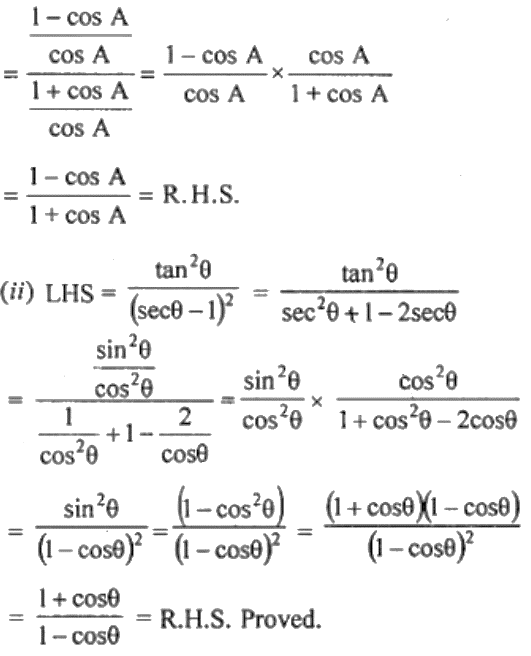Guru

# (i) (sec A – 1)/(sec A + 1) = (1 – cos A)/(1 + cos A) (ii) tan2 θ/ (sec θ – 1)2 = (1 + cos θ)/ (1 – cos θ) (iii) (1 + tan A)2 + (1 – tan A)2 = 2 sec2 A (iv) sec2 A + cosec2 A = sec2 A. cosec2 A

• 0

This question has been taken from the Book- ML Aggarwal
Board- ICSE
Publication- Avichal
Chapter- Trigonometric Identities
Chapter number-18
We have to prove that

(i) (sec A – 1)/(sec A + 1) = (1 – cos A)/(1 + cos A) (ii) tan2 θ/ (sec θ – 1)2 = (1 + cos θ)/ (1 – cos θ) (iii) (1 + tan A)2 + (1 – tan A)2 = 2 sec2 A (iv) sec2 A + cosec2 A = sec2 A. cosec2 A

Class10th, chapter 18, trigonometric identities, ques no. 16

Share

1. Solution:(iii) L.H.S. = (1 + tan A)2 + (1 – tan A)2

= 1 + 2 tan A + tan2 A + 1 – 2 tan A + tan2 A

= 2 + 2 tan2 A

= 2(1 + tan2 A) [As 1 + tan2 A = sec2 A]

= 2 sec2 A

= R.H.S.

(iv) L.H.S = secA + cosec2 A

= 1/cos2 A + 1/sin2 A

= (sin2 A + cos2 A)/ (sin2 A cos2 A)

= 1/ (sin2 A cos2 A)

= sec2 A cosec2 A = R.H.S

• 0